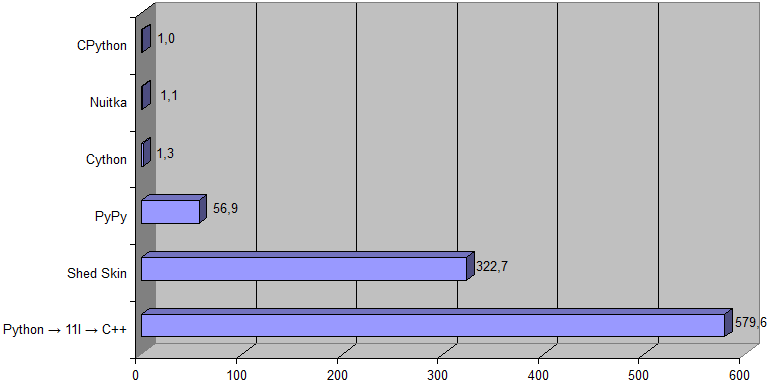The Python → 11l → C++ transpiler is a union of 2 transpilers:
1. The Python → 11l transpiler, which translates Python code into 11l.
2. The 11l → C++ transpiler, which translates 11l code into C++ [which is then compiled into machine code].

In a sense, the 11l programming language acts as an intermediate language, but since it is very similar and close to the Python language (albeit semantically, not syntactically), it is generally not required to study it specifically.

Also it should be noted that this transpiler generates a human-readable code, which simplifies debugging of the generated program.

 Performance Here is the results for pqmarkup to HTML converter (as an input data the source of this article is taken):(Shed Skin is not listed here because it does not support nested functions.) [Here is an archive with testing program used (Python 3.6 or higher is required, and following Python packages: pywin32, cython).] This is the results for a Prime number problem (with K = 1000000):Here is a corresponding Python source code span.keyword {color: #0000FF; font-weight: bold;} span.identifier {color: #00009F;} span.string-literal {color: #800000;} span.numeric-literal {color: #008000;} span.constant {color: #008000;} span.comment {color: #808080;} ```import math k = int(input()) n = k * 17 primes = [True] * n primes = primes = False for i in range(2, int(math.sqrt(n)) + 1): if not primes[i]: continue for j in range(i * i, n, i): primes[j] = False for i in range(n): if primes[i]: if k == 1: print(i) break k -= 1 ``` And this code {… ```def is_right_triangle(x1, y1, x2, y2): a = x1**2 + y1**2 b = x2**2 + y2**2 c = (x2 - x1)**2 + (y2 - y1)**2 return (a + b == c) or (b + c == a) or (c + a == b) LIMIT = 51 ans = 0 for x1 in range(LIMIT): for y1 in range(LIMIT): for x2 in range(LIMIT): for y2 in range(LIMIT): # For uniqueness, ensure that (x1,y1) has a larger angle than (x2,y2) if y2 * x1 < y1 * x2 and is_right_triangle(x1, y1, x2, y2): ans += 1 print(ans) ``` (Based on this solution.) } for solving problem #91 of the Project Euler is ~500 times faster (the Python → 11l → C++ transpiler vs CPython):Download Here is the latest version of the Python → 11l → C++ transpiler: 11l.tar.xz . Usage: Unpack archive to whatever directory you want. Open Terminal/cmd. Run command: `\11l ` on Windows, or `/11l ` on Linux. Having problems with transpiling your Python program? Please read this guide. And if you haven't found a solution there, then write at the forum.